Students can Download Accountancy Chapter 5 Admission of a Partner Questions and Answers, Notes Pdf, Samacheer Kalvi 12th Accountancy Book Solutions Guide Pdf helps you to revise the complete Tamilnadu State Board New Syllabus and score more marks in your examinations.

## Tamilnadu Samacheer Kalvi 12th Accountancy Solutions Chapter 5 Admission of a Partner

### Samacheer Kalvi 12th Accountancy Admission of a Partner Text Book Back Questions and Answers

12th Accountancy 5th Chapter Solutions Question 1.
Revaluation A/c is a ……………..
(a) Real A/c
(b) Nominal A/c
(c) Personal A/c
(d) Impersonal A/c
(b) Nominal A/c

12th Accountancy Chapter 5 Solutions Question 2.
On revaluation, the increase in the value of assets leads to ……………..
(a) Gain
(b) Loss
(c) Expense
(d) None of these
(a) Gain

Class 12 Accountancy Chapter 5 Solutions Question 3.
The profit or loss on revaluation of assets and liabilities is transferred to the capital account of ……………..
(a) The old partners
(b) The new partner
(c) All the partners
(d) The Sacrificing partners
(a) The old partners

12th Accountancy Chapter 5 Question 4.
If the old profit sharing ratio is more than the new profit sharing ratio of a partner, the difference is called ……………..
(a) Capital ratio
(b) Sacrificing ratio
(c) Gaining ratio
(d) None of these
(b) Sacrificing ratio

12th Accounts Chapter 5 Solutions Question 5.
At the time of admission, the goodwill brought by the new partner may be credited to the capital accounts of ……………..
(a) all the partners
(b) the old partners
(c) the new partner
(d) the sacrificing partners
(d) the sacrificing partners

Accountancy Class 12 Chapter 5 Solutions Question 6.
Which of the following statements is not true in relation to admission of a partner?
(a) Generally mutual rights of the partners change
(b) The profits and losses of the previous years are distributed to the old partners
(c) The firm is reconstituted under a new agreement
(d) The existing agreement does not come to an end
(d) The existing agreement does not come to an end

Accountancy Chapter 5 Class 12 Question 7.
Match List I with List II and select the correct answer using the codes given below: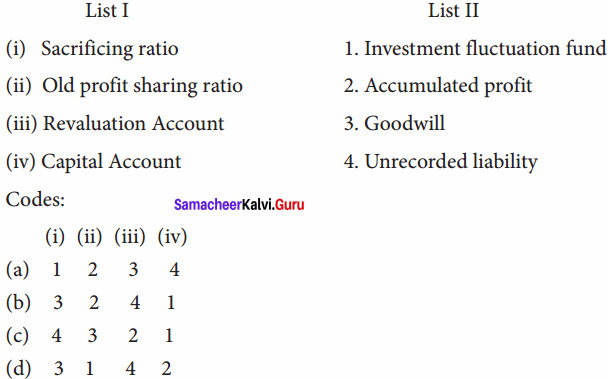(b) 3 2 4 1

Class 12th Accounts Chapter 5 Solutions Question 8.
Select the odd one out:
(a) Revaluation profit
(b) Accumulated loss
(c) Goodwill brought by new partner
(d) Investment fluctuation fund
(c) Goodwill brought by new partner

12th Accountancy 5th Chapter Solutions Pdf Question 9.
James and Kamal are sharing profits and losses in the ratio of 5:3. They admit Sunil as a partner giving him 1/5 share of profits. Find out the sacrificing ratio.
(a) 1:3
(b) 3:1
(c) 5:3
(d) 3:5
(c) 5:3

Accounts Chapter 5 Class 12 Solutions Question 10.
Balaji and Kamalesh are partners sharing profits and losses in the ratio of 2:1. They admit Yogesh into partnership. The new profit sharing ratio between Balaji, Kamalesh and Yogesh is agreed to 3:1:1. Find the sacrificing ratio between Balaji and Kamalesh.
(a) 1:3
(b) 3:1
(c) 2:1
(d) 1:2
(d) 1:2

Samacheer Kalvi Guru 12th Accountancy Question 1.
What is meant by revaluation of assets and liabilities?
When a partner is admitted into the partnership the assets and liabilities are revealed as the current value may differ from the book value. Determination of
current values of assets and liabilities is called revaluation of assets and liabilities.

Chapter 5 Accounts Class 12 Question 2.
How are accumulated profits and losses distributed among the partners at the time of admission of a new partner?
Profits and losses of previous years which are not distributed to the partners are called accumulated profit and losses. This belong to the old partners and hence these should be distributed to the old partners in the old profit sharing ratio.

Chapter 5 Accounts Class 12 Solutions Question 3.
What is sacrificing ratio?
Sacrificing ratio is the proportion of the profit which is sacrificed or foregone by the old partners in favour of the new partner. The purpose of finding the sacrificing ratio is to share the goodwill brought in by the new partner.
Sacrifice Ratio = Old share – New share

Chapter 5 Class 12 Accounts Question 4.
Give the journal entry for writing off existing goodwill at the time of admission of a new partner.
Journal Entry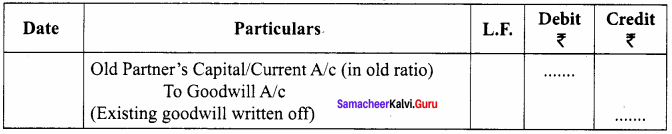Std 12 Account Chapter 5 Question 5.
State whether the following will be debited or credited in the revaluation account.

1. Depreciation on assets
2. Unrecorded liability
3. Provision for outstanding expenses
4. Appreciation of assets

1. Depreciation on assets – Debited
2. Unrecorded liability – Debited
3. Provision for outstanding expenses – Debited
4. Appreciation of assets – Debited

12th Accountancy 3rd Chapter Solutions Question 1.
What are the adjustments required at the time of admission of a partner?
The following adjustments are necessary at the time of admission of a partner.

1. Distribution of accumulated profits, reserves and losses
2. Revaluation of assets and liabilities
3. Determination of new profit sharing ratio and sacrificing ratio
5. Adjustment of capital on the basis of new profit sharing ratio

12th Accountancy 6th Chapter Solutions Question 2.
What are the journal entries to be passed on revaluation of assets and liabilities?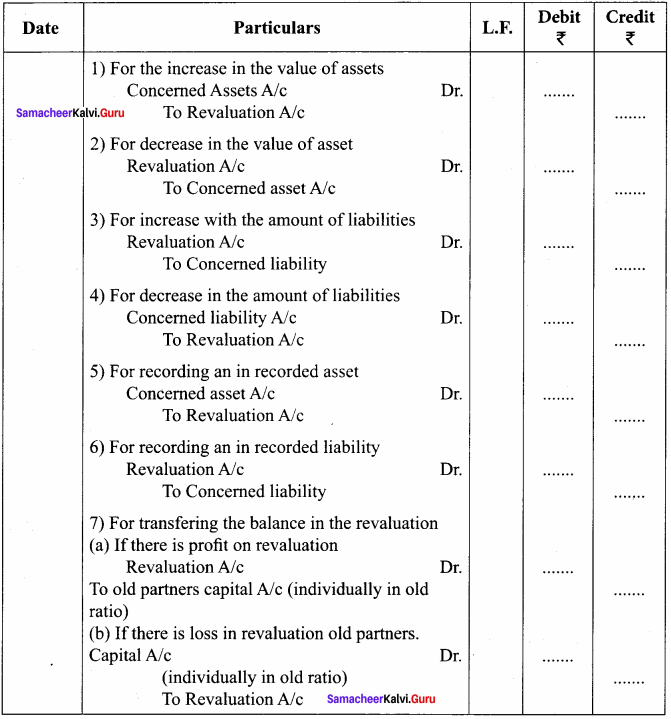Class 12 Accountancy Chapter 5 Question 3.
Write a short note on accounting treatment of goodwill.
1. For the goodwill brought in cash credited to old partner’s capital account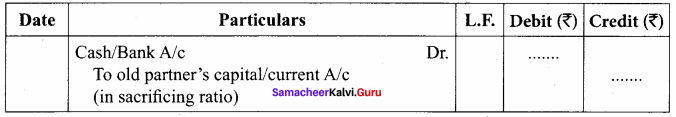2. For the goodwill brought in kind (in the form of asset) credited to old partner’s capital account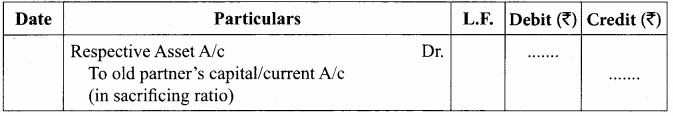3. For withdrawal of cash received for goodwill by the old partners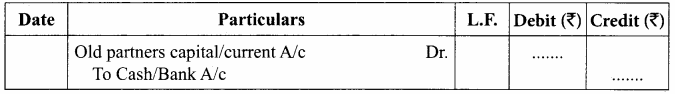III. Exercises

Chapter 5 Admission Of A Partner Question 1.
Arul and Anitha are partners sharing profits and losses in the ratio of 4 : 3. On 31.3.2018, Ajay was admitted as a partner. On the date of admission, the book of the firm showed a general reserve of ₹ 42,000. Pass the journal entry to distribute the general reserve.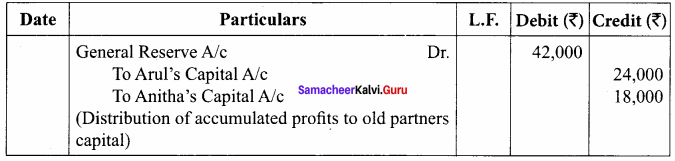Ch 5 Accounts Class 12 Question 2.
Anjali and Nithya are partners of a firm sharing profits and losses in the ratio of 5 : 3. They admit Pramila on 1.1.2018. On that date, their balance sheet showed accumulated loss of ₹ 40,000 on the asset side of the balance sheet. Give the journal entry to transfer the accumulated loss on admission.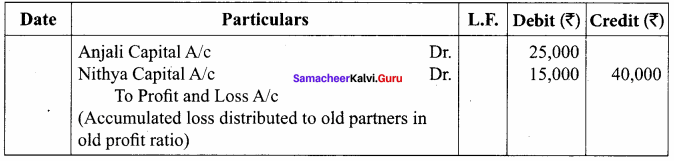Samacheer Kalvi 12th Accountancy Solutions Chapter 1 Question 3.
Oviya and Kavya are partners in a firm sharing profits and losses in the ratio of 5:3. They admit Agalya into the partnership. Their balance sheet as on 31st March, 2019 is as follows:
Balance Sheet as on 31st March 2019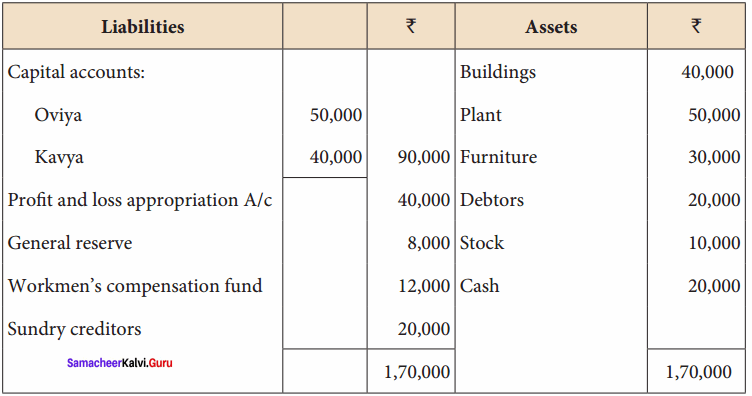Pass journal entry to transfer the accumulated profits and reserve on admission.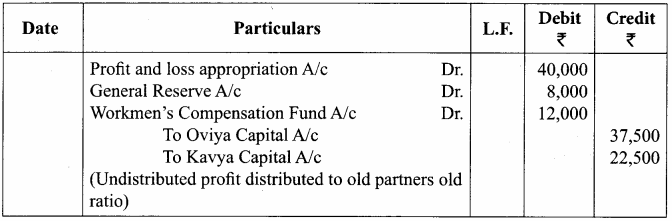Question 4.
Hari, Madhavan and Kesavan are partners, sharing profits and losses in the ratio of 5:3:2. As from 1st April 2017, Vanmathi is admitted into the partnership and the new profit sharing ratio is decided as 4:3:2:1. The following adjustments are to be made.

1. Increase the value of premises by ₹ 60,000.
2. Depreciate stock by ₹ 5,000, furniture by ₹ 2,000 and machinery by ₹ 2,500.
3. Provide for an outstanding liability of ₹ 500.

Pass journal entries and prepare revaluation account.
Journal Entries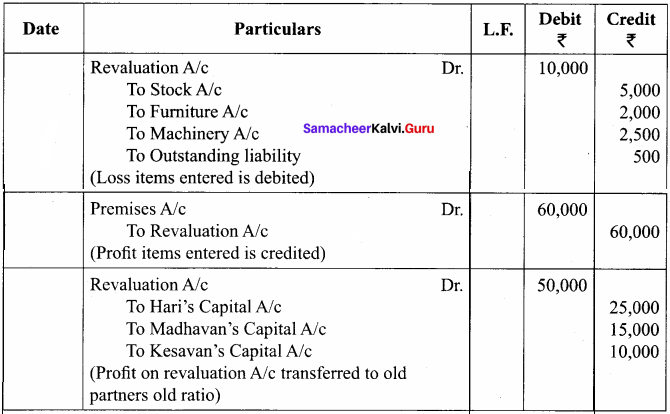Revaluation Account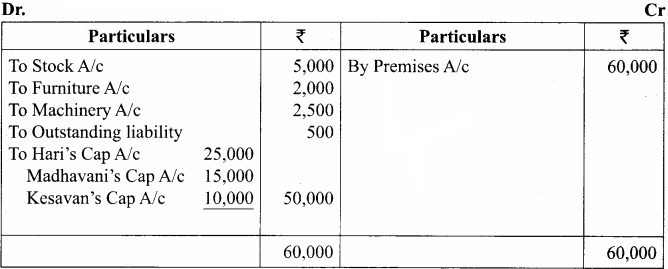Question 5.
Seenu and Siva are partners sharing profits and losses in the ratio of 5:3. In the view of Kowsalya admission, they decided

1. To increase the value of building by ₹ 40,000.
2. To bring into record investments at ₹ 10,000, which have not so far been brought into account.
3. To decrease the value of machinery by ₹ 14,000 and furniture by ₹ 12,000.
4. To write off sundry creditors by ₹ 16,000.

Pass journal entries and prepare revaluation account.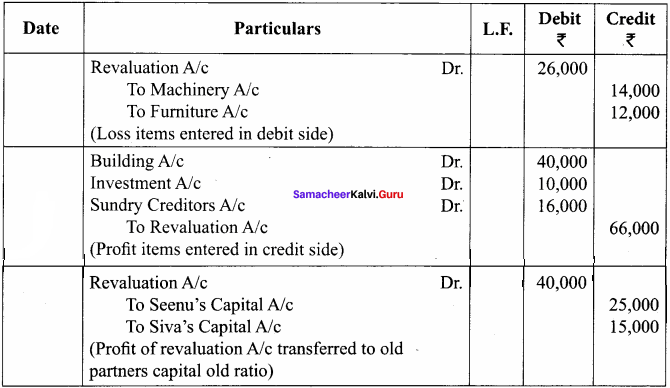Revaluation Account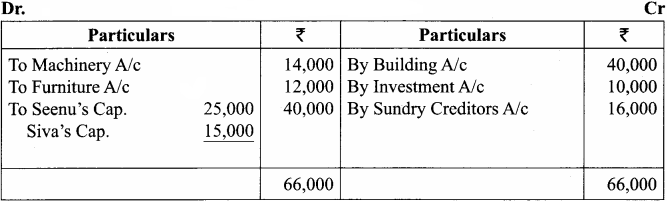Question 6.
Sai and Shankar are partners, sharing profits and losses in the ratio of 5 : 3. The firm’s balance sheet as on 31st December, 2017, was as follows: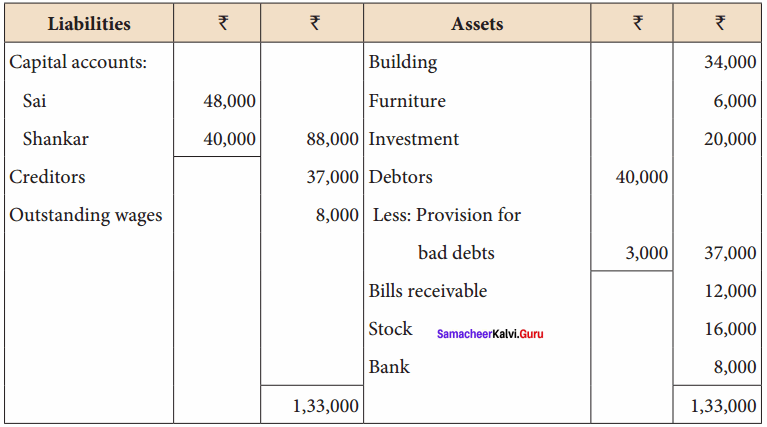On 31st December, 2017 Shanmugam was admitted into the partnership for 1/4 share of profit with ₹ 12,000 as capital subject to the following adjustments.

1. Furniture is to be revalued at ₹ 5,000 and building is to be revalued at ₹ 50,000
2. Provision for doubtful debts is to be increased to ₹ 5,500
3. An unrecorded investment of ₹ 6,000 is to be brought into account
4. An unrecorded liability ₹ 2,500 has to be recorded now

Pass journal entries and prepare Revaluation Account and capital account of partners after admission.
Journal Entries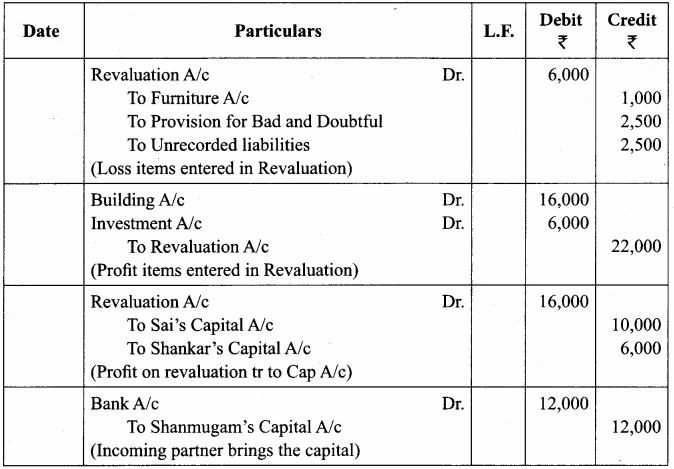Revaluation Account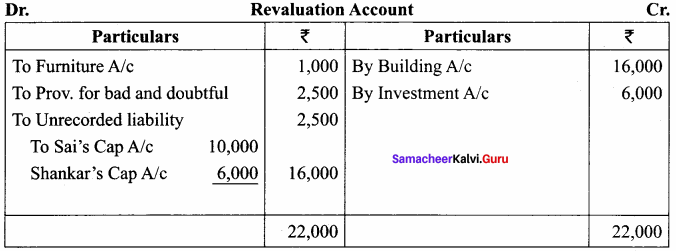Capital Account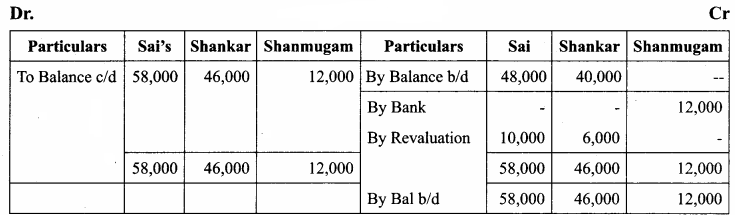Question 7.
Amal and Vimal are partners in a firm sharing profits and losses in the ratio of 7 : 5. Their balance sheet as on 31st March, 2019, is as follows: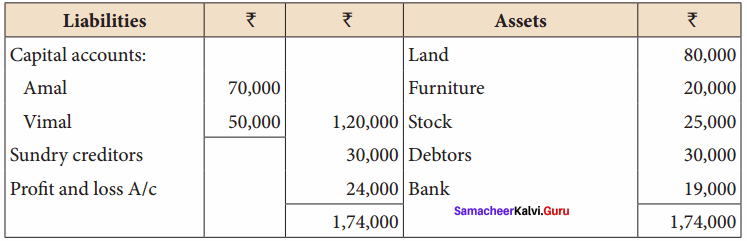Nirmal is admitted as a new partner on 1.4.2018 by introducing a capital of 30,000 for 1/3 share in the future profit subject to the following adjustments.

1. Stock to be depreciated by ₹ 5,000
2. Provision for doubtful debts to be created for ₹ 3,000
3. Land to be appreciated by ₹ 20,000

Prepare revaluation account and capital account of partners after admission.
Revaluation Account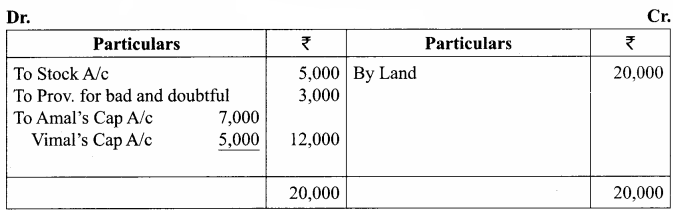Capital Account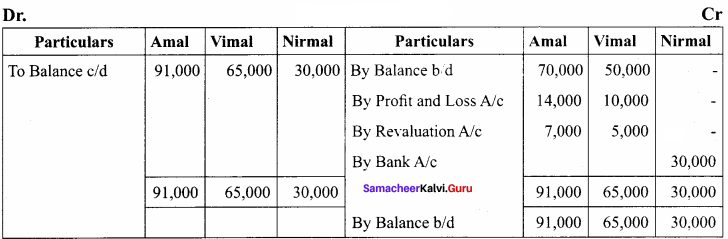Question 8.
Praveena and Dhanya are partners sharing profits in the ratio of 7 : 3. They admit Malini into the firm. The new ratio among Praveena, Dhanya and Malini is 5 : 2 : 3. Calculate the sacrificing ratio.
Sacrificing Ratio = Old Ratio – New Ratio
Praveena = $$\frac { 7 }{ 10 }$$ – $$\frac { 5 }{ 10 }$$ = $$\frac { 2 }{ 10 }$$
Dhanya = $$\frac { 3 }{ 10 }$$ – $$\frac { 2 }{ 10 }$$ = $$\frac { 1 }{ 10 }$$
Sacrificing Ratio = 2 : 1

Question 9.
Ananth and Suman are partners sharing profits and losses in the ratio of 3 : 2. They admit Saran for 1/5 share, which he acquires entirely from Ananth. Find out the new profit sharing ratio and sacrificing ratio.
New Profit Sharing Ratio:
Ananth = $$\frac { 3 }{ 5 }$$ – $$\frac { 1 }{ 5 }$$ = $$\frac { 2 }{ 5 }$$
Suman = $$\frac { 2 }{ 5 }$$ – = $$\frac { 2 }{ 5 }$$
Ananth = $$\frac { 1}{ 5 }$$ – = $$\frac { 1 }{ 5 }$$
New Profit sharing Ratio = 2 : 2 : 1
Sacrificing Ratio = 1 : 0

Question 10.
Raja and Ravi are partners, sharing profits in the ratio of 3 : 2. They admit Ram for 1/4 share of the profit. He takes 1/20 share from Raja and 4/20 from Ravi. Calculate the new profit sharing ratio and sacrificing ratio.
New Profit Sharing Ratio = Old Ratio – Sacrificing Ratio
Raja = $$\frac { 3 }{ 5 }$$ – $$\frac { 1 }{ 20 }$$ = $$\frac { 12 – 1 }{ 20 }$$ = $$\frac { 11 }{ 20 }$$
Ravi = $$\frac { 2 }{ 5 }$$ – $$\frac { 4 }{ 20 }$$ = $$\frac { 8 – 4 }{ 20 }$$ = $$\frac { 4 }{ 20 }$$
Ram = $$\frac { 1 }{ 20 }$$ + $$\frac { 4 }{ 20 }$$ = $$\frac { 5 }{ 20 }$$
New Profit sharing Ratio = 11 : 4 : 5
Sacrificing Ratio = $$\frac { 1 }{ 20 }$$ : $$\frac { 4 }{ 20 }$$

Question 11.
Vimala and Kamala are partners, sharing profits and losses in the ratio of 4:3. Vinitha enters into the partnership and she acquires 1/14 from Vimala and 1/14 from Kamala. Find out the new profit sharing ratio and sacrificing ratio.
New Profit Sharing Ratio = Old Ratio – Sacrificing Ratio
Vimala = $$\frac { 4 }{ 7 }$$ – $$\frac { 1 }{ 14 }$$ = $$\frac { 8 – 1 }{ 14 }$$ = $$\frac { 7 }{ 14 }$$
Kamala = $$\frac { 3 }{ 7 }$$ – $$\frac { 1 }{ 14 }$$ = $$\frac { 6 – 1 }{ 14 }$$ = $$\frac { 5 }{ 14 }$$
Vinitha = $$\frac { 1 }{ 14 }$$ + $$\frac { 1 }{ 14 }$$ = $$\frac { 2 }{ 14 }$$
New Profit Sharing Ratio = 7 : 5 : 2
Sacrificing Ratio = 1 : 1

Question 12.
Govind and Gopal are partners in a firm sharing profits in the ratio of 5 : 4. They admit Rahim as a partner. Govind surrenders 2/9 of his share in favour of Rahim. Gopal surrenders 1/9 of his share in favour of Rahim. Calculate the new profit sharing ratio and sacrificing ratio.
New Profit Sharing Ratio:
Govind = $$\frac { 5 }{ 9 }$$ x $$\frac { 2 }{ 9 }$$ = $$\frac { 10 }{ 81 }$$ ; $$\frac { 5 }{ 9 }$$ – $$\frac { 10 }{ 81 }$$ = $$\frac { 45 – 10 }{ 81 }$$ = $$\frac { 35 }{ 81 }$$
Gopal = $$\frac { 4 }{ 9 }$$ x $$\frac { 1 }{ 9 }$$ = $$\frac { 4 }{ 81 }$$ ; $$\frac { 3 }{ 8 }$$ – $$\frac { 3 }{ 64 }$$ = $$\frac { 24 – 3 }{ 64 }$$ = $$\frac { 21 }{ 64 }$$
Rahim = $$\frac { 2 }{ 9 }$$ + $$\frac { 1 }{ 9 }$$ = $$\frac { 3 }{ 9 }$$ ; $$\frac { 10 }{ 81 }$$ + $$\frac { 4 }{ 81 }$$ = $$\frac { 14 }{ 81 }$$
New Profit Sharing Ratio = 35 : 32 : 14
Sacrificing Ratio = $$\frac { 10 }{ 81 }$$ : $$\frac { 4 }{ 81 }$$ = 5 : 2

Question 13.
Prema and Chandra share profits in the ratio of 5:3. Hema is admitted as a partner. Prema surrendered 1/8 of her share and Chandra surrendered 1/8 of her share in favour of Hema. Calculate the new profit sharing ratio and sacrificing ratio.
New Profit Sharing Ratio:
Prema = $$\frac { 5 }{ 8 }$$ x $$\frac { 1 }{ 8 }$$ = $$\frac { 5 }{ 64 }$$ ; $$\frac { 5 }{ 8 }$$ – $$\frac { 5 }{ 64 }$$ = $$\frac { 40 – 5 }{ 64 }$$ = $$\frac { 35 }{ 64 }$$
Chandra = $$\frac { 3 }{ 8 }$$ x $$\frac { 1 }{ 8 }$$ = $$\frac { 3 }{ 64 }$$ ; $$\frac { 3 }{ 8 }$$ – $$\frac { 3 }{ 64 }$$ = $$\frac { 24 – 3 }{ 64 }$$ = $$\frac { 21 }{ 64 }$$
Hema = $$\frac { 5 }{ 64 }$$ + $$\frac { 3 }{ 64 }$$ = $$\frac { 8 }{ 64 }$$
New Profit Sharing Ratio = 35 : 21 : 8
Sacrificing Ratio = 5 : 3

Question 14.
Karthik and Kannan are equal partners. They admit Kailash with 1/4 share of the profit. Kailash acquired his share from old partners in the ratio of 7:3. Calculate the new profit sharing ratio and sacrificing ratio.
New Profit Sharing Ratio:
Karthik = $$\frac { 1 }{ 4 }$$ x $$\frac { 7 }{ 10 }$$ = $$\frac { 7 }{ 40 }$$ ; $$\frac { 1 }{ 2 }$$ – $$\frac { 7 }{ 40 }$$ = $$\frac { 20 – 7 }{ 40 }$$ = $$\frac { 13 }{ 40 }$$
Kannan = $$\frac { 1 }{ 4 }$$ x $$\frac { 3 }{ 10 }$$ = $$\frac { 3 }{ 40 }$$ ; $$\frac { 1 }{ 2 }$$ – $$\frac { 3 }{ 40 }$$ = $$\frac { 20 – 3 }{ 40 }$$ = $$\frac { 17 }{ 40 }$$
Kailash = $$\frac { 1 }{ 4 }$$ x 10 = $$\frac { 10 }{ 40 }$$
New Profit Sharing Ratio = 13 : 17 : 10
Sacrificing Ratio = 7 : 3

Question 15.
Selvam and Senthil are partners sharing profit in the ratio of 2:3. Siva is admitted into the firm with 1/5 share of profit. Siva acquires equally from Selvam and Senthil. Calculate the new profit sharing ratio and sacrificing ratio.
New Profit Sharing Ratio:
Siva’s share = $$\frac { 1 }{ 5 }$$ x $$\frac { 1 }{ 2 }$$ = $$\frac { 1 }{ 10 }$$
Selvam’s share = $$\frac { 2 }{ 5 }$$ – $$\frac { 1 }{ 10 }$$ = $$\frac { 4 – 1 }{ 10 }$$ = $$\frac { 3 }{ 10 }$$
Senthil’s share = $$\frac { 3 }{ 5 }$$ – $$\frac { 1 }{ 10 }$$ = $$\frac { 6 – 1 }{ 10 }$$ = $$\frac { 5 }{ 10 }$$
Siva’s share = $$\frac { 1 }{ 10 }$$ + $$\frac { 1 }{ 10 }$$ = $$\frac { 2 }{ 10 }$$
New Profit sharing Ratio = 3 : 5 : 2
Sacrificing Ratio = 1 : 1

Question 16.
Mala and Anitha are partners, sharing profits and losses in the ratio of 3 : 2. Mercy is admitted into the partnership with 1/5 share in the profits. Calculate new profit sharing ratio and sacrificing ratio.
Calculate New Profit Sharing Ratio = 1 – $$\frac { 1 }{ 5 }$$ = $$\frac { 4 }{ 5 }$$
Mala = $$\frac { 3 }{ 5 }$$ x $$\frac { 4 }{ 5 }$$ = $$\frac { 12 }{ 25 }$$
Anitha = $$\frac { 2 }{ 5 }$$ x $$\frac { 4 }{ 5 }$$ = $$\frac { 8 }{ 25 }$$
Mercy = $$\frac { 1 }{ 5 }$$ x 5 = $$\frac { 5 }{ 25 }$$
New Profit Sharing Ratio = 12 : 8 : 5
Sacrificing Ratio = Old share – New share
Mala = $$\frac { 3 }{ 5 }$$ – $$\frac { 12 }{ 25 }$$ = $$\frac { 15 – 12}{ 25 }$$ = $$\frac { 3 }{ 25 }$$
Anitha = $$\frac { 2 }{ 5 }$$ – $$\frac { 8 }{ 25 }$$ = $$\frac { 2 }{ 25 }$$
Sacrificing Ratio = 3 : 2

Question 17.
Ambika, Dharani and Padma are partners in a firm sharing profits in the ratio of 5:3:2. They admit Ramya for 25% profit. Calculate the new profit sharing ratio and sacrificing ratio.
Ramya share = 25% = 100% – 25% = 75% = $$\frac { 3 }{ 4 }$$
Ambika’s share = $$\frac { 5 }{ 10 }$$ x $$\frac { 3 }{ 4 }$$ = $$\frac { 15 }{ 40 }$$
Dharani’s share = $$\frac { 3 }{ 10 }$$ x $$\frac { 3 }{ 4 }$$ = $$\frac { 9 }{ 40 }$$
Padma’s share = $$\frac { 2 }{ 10 }$$ x $$\frac { 3 }{ 4 }$$ = $$\frac { 6 }{ 40 }$$
Mercy’s share
New Profit Sharing Ratio
Sacrificing Ratio = Old share – New share
Ambika’s share = $$\frac { 5 }{ 10 }$$ – $$\frac { 15 }{ 40 }$$ = $$\frac { 20 – 15 }{ 40 }$$ = $$\frac { 5 }{ 40 }$$
Dharani’s share = $$\frac { 3 }{ 10 }$$ – $$\frac { 9 }{ 40 }$$ = $$\frac { 12 – 9 }{ 40 }$$ = $$\frac { 3 }{ 40 }$$
Padma’s share = $$\frac { 2 }{ 10 }$$ – $$\frac { 6 }{ 40 }$$ = $$\frac { 8 – 6 }{ 40 }$$ = $$\frac { 2 }{ 40 }$$
Sacrificing Ratio = 5 : 3 : 2

Question 18.
Aparna and Priya are partners who share profits and losses in the ratio of 3 : 2. Brindha joins the firm for 1/5 share of profits and brings in cash for her share of goodwill of ₹ 10,000. Pass necessary journal entry for adjusting goodwill on the assumption that the fluctuating capital method is followed and the partners withdraw the entire amount of their share of goodwill.
Journal Entries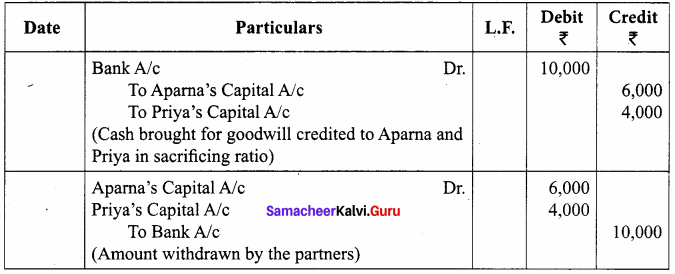Question 19.
Deepak, Senthil and Santhosh are partners sharing profits and losses equally. They admit Jerald into partnership for 1/3 share in future profits. The goodwill of the firm is valued at ₹ 45,000 and Jerald brought cash for his share of goodwill. The existing partners withdraw half of the amount of their share of goodwill. Pass necessary journal entries for adjusting goodwill on the assumption that the fluctuating capital method is followed.
Journal Entries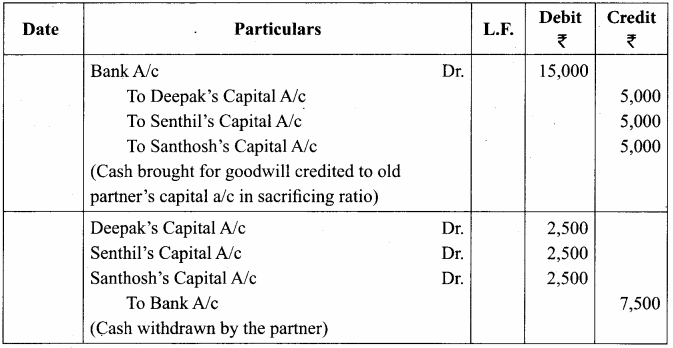Jerald’s Share of goodwill = ₹ 45000 x 1/3 = ₹ 1,500
As the sacrifice made by the existing partners is not mentioned. It is assumed that they sacrifice in their old share profit ratio = 1 : 1 : 1.
Therefore, Sacrificing ratio = 1 : 1 : 1.

Question 20.
Malathi and Shobana are partners sharing profits and losses in the ratio of 5 : 4. They admit Jayasri into partnership for 1/3 share of profit. Jayasri pays cash ₹ 6,000 towards her share of goodwill. The new ratio is 3 : 2 : 1. Pass necessary journal entry for adjusting goodwill on the assumption that the fixed capital method is followed.
Adjusting Goodwill: Old share – New Share
Malathi’s share = $$\frac { 5 }{ 9 }$$ – $$\frac { 3 }{ 6 }$$ = $$\frac { 10 – 9 }{ 18 }$$ = $$\frac { 1 }{ 18 }$$
Shobana’s share = $$\frac { 4 }{ 9 }$$ – $$\frac { 2 }{ 6 }$$ = $$\frac { 8 – 6 }{ 18 }$$ = $$\frac { 2 }{ 18 }$$

Question 21.
Anu and Arul were partners in a firm sharing profits and losses in the ratio of 4 : 1. They have decided to admit Mano into the firm for 2/5 share of profits. The goodwill of the firm on the date of admission was valued at ₹ 25,000. Mano is not able to bring in cash for his share of goodwill. Pass necessary journal entry for goodwill on the assumption that the fluctuating capital method is followed.
Mano’s shares: 25000 x $$\frac { 2 }{ 5 }$$ = Rs 10000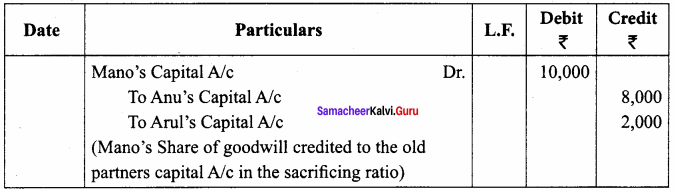Question 22.
Varun and Barath are partners sharing profits and losses 5 : 4. They admit Dhamu into partnership. The new profit sharing ratio is agreed at 1 : 1 : 1. Dhamu’s share of goodwill is valued at ₹ 15,000 of which he pays ₹ 10,000 in cash. Pass necessary journal entries for adjustment of goodwill on the assumption that the fluctuating capital method is followed.
Dhamu’s share of goodwill against sacrificing ratio:
Sacrificing Ratio = Old share – New share
Varun = $$\frac { 5 }{ 9 }$$ – $$\frac { 1 }{ 3 }$$ = $$\frac { 5 – 3 }{ 9 }$$ = $$\frac { 2 }{ 9 }$$
Barath = $$\frac { 4 }{ 9 }$$ – $$\frac { 1 }{ 3 }$$ = $$\frac { 4 – 3 }{ 9 }$$ = $$\frac { 1 }{ 9 }$$
Goodwill value = $$\frac { 15000 }{ 3 }$$ = ₹ 5000.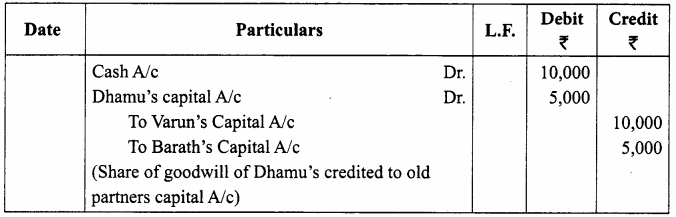Question 23.
Sam and Jose are partners in a firm sharing profits and losses in the ratio of 3 : 2. On 1st April 2018, they admitted Joel as a partner. On the date of Joel’s admission, goodwill appeared in the books of the firm at ₹ 30,000. By assuming fluctuating capital method, pass the necessary journal entry if the partners decide to

1. write off the entire amount of existing goodwill
2. write off ₹ 20,000 of the existing goodwill.

Journal Entries
(a) Write off the entire amount of existing goodwill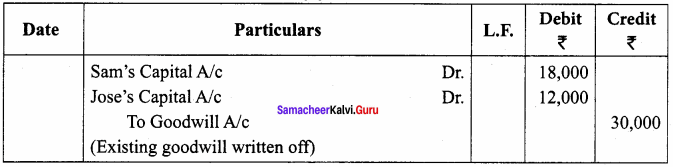(b) Write off ₹ 20,000 of the existing goodwill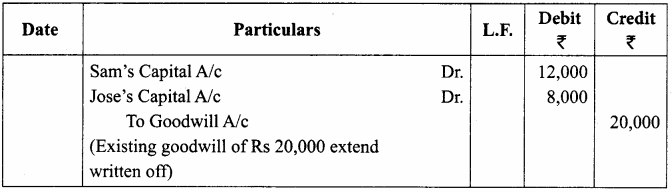Question 24.
Raj an and Selva are partners sharing profits and losses in the ratio of 3:1. Their balance sheet as on 31st March 2017 is as under: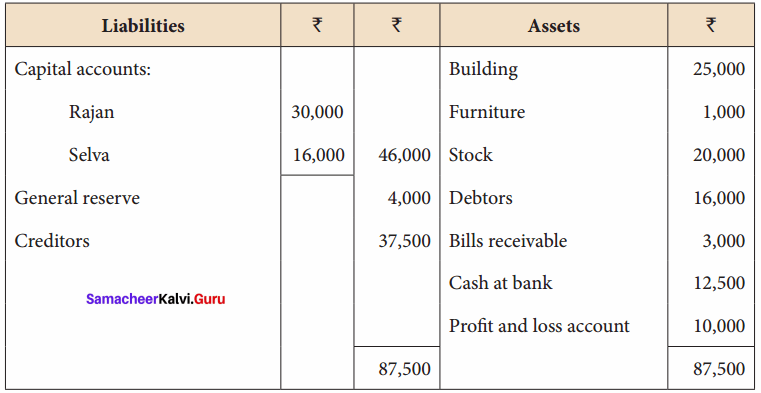On 1.4.2017, they admit Ganesan as a new partner on the following arrangements:

1. Ganesan brings ₹ 10,000 as capital for 1/5 share of profit.
2. Stock and furniture is to be reduced by 10%, a reserve of 5% on debtors for doubtful debts is to be created.
3. Appreciate buildings by 20%.

Prepare revaluation account, partners’ capital account and the balance sheet of the firm after admission.
Revaluation Account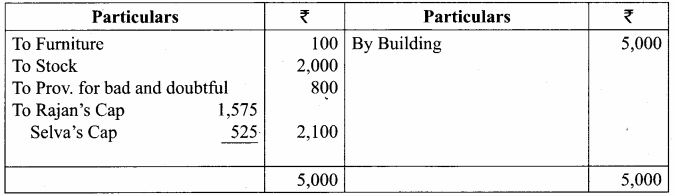Capital Account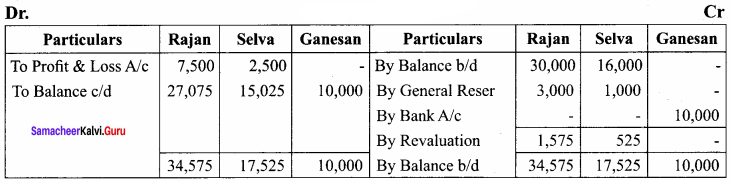Balance Sheet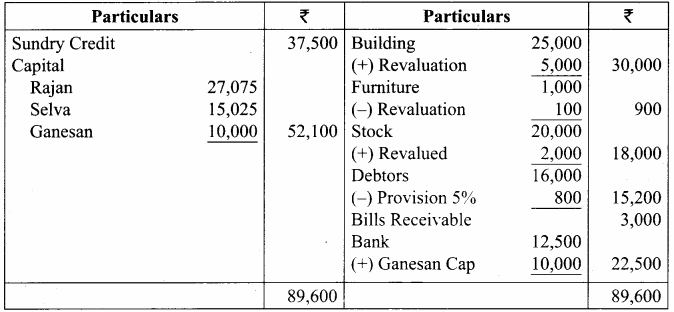Question 25.
Sundar and Suresh are partners sharing profits in the ratio of 3:2. Their balance sheet as on 1st January, 2017 was as follows: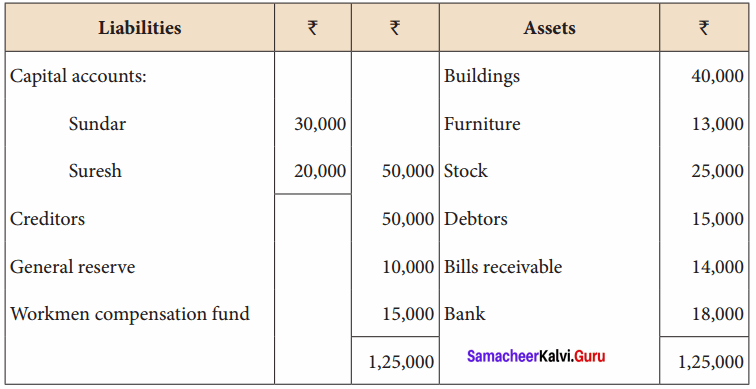They decided to admit Sugumar into partnership for 1/4 share in the profits on the following terms:

1. Sugumar has to bring in ₹ 30,000 as capital. His share of goodwill is valued at ₹ 5,000. He could not bring cash towards goodwill.
2. That the stock be valued at ₹ 20, 000.
3. That the furniture be depreciated by ₹ 2,000.
4. That the value of building be depreciated by 20%.

Prepare necessary ledger accounts and the balance sheet after admission.
Revaluation Account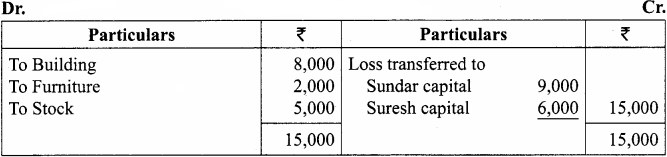Capital Account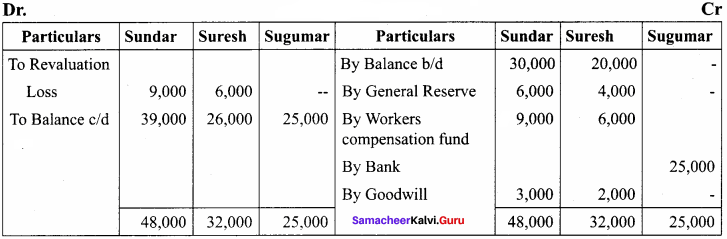Balance Sheet as on 31.12.17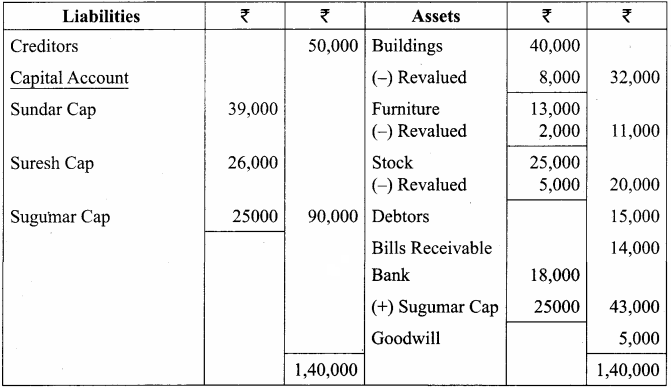Question 26.
The following is the balance sheet of Janies and Justina as on 1.1.2017. They share the profits and losses equally.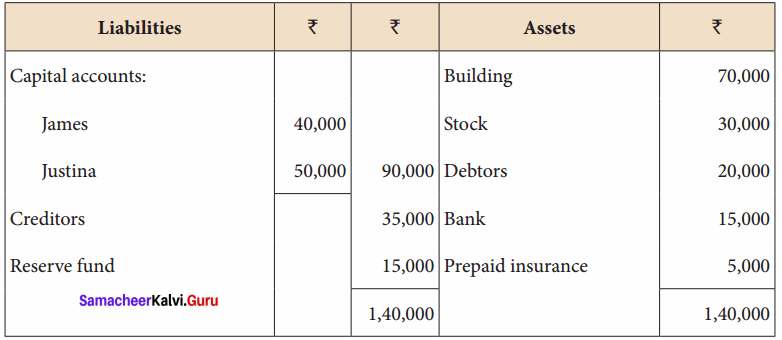On the above date, Balan is admitted as a partner with 1/5 share in future profits. Following are the terms for his admission:

1. Balan brings ₹ 25,000 as capital.
2. His share of goodwill is ₹ 10,000 and he brings cash for it.
3. The assets are to be valued as under:

Building ₹ 80,000; Debtors ₹ 18,000; Stock ₹ 33,000 Prepare necessary ledger accounts and the balance sheet after admission.
Revaluation Account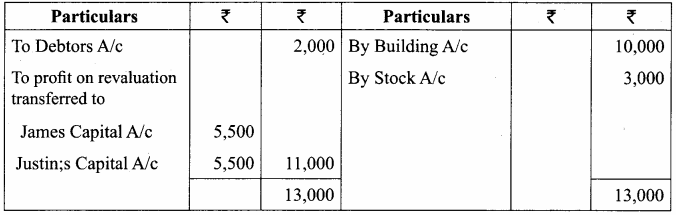Capital Account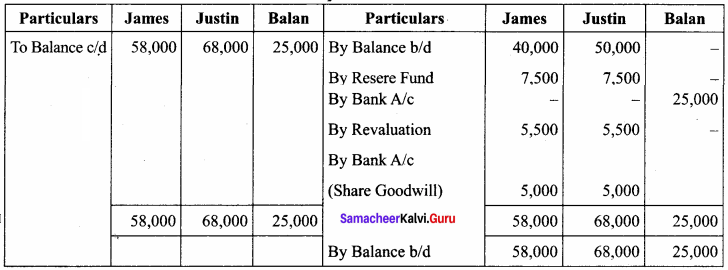Cash Account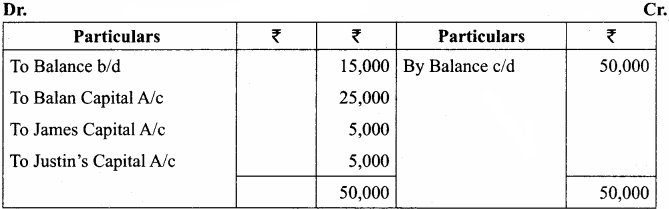Balance Sheet as on 01.01.2017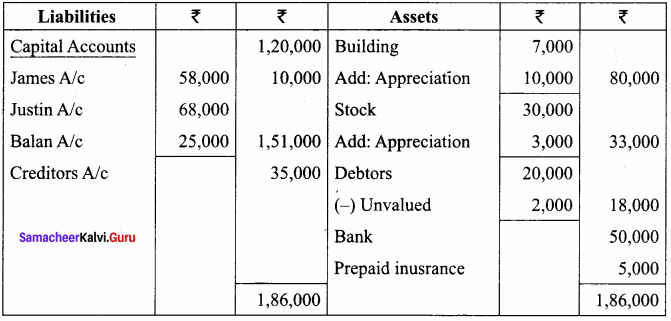Question 27.
Anbu and Shankar are partners in a business sharing profits and losses in the ratio of 7 : 5. The balance sheet of the partners on 31.03.2018 is as follows: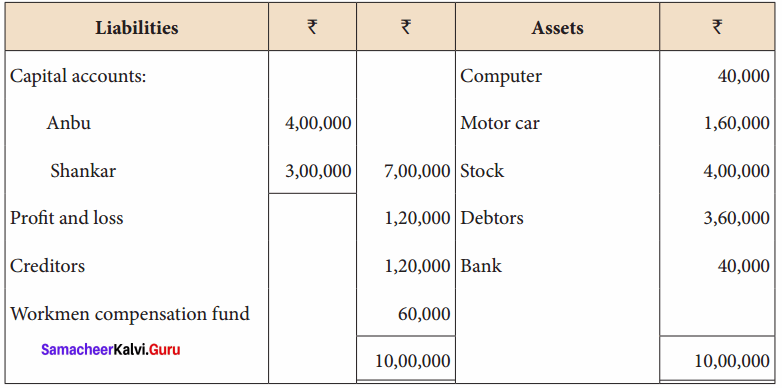Rajesh is admitted for 1/5 share on the following terms:

1. Goodwill of the firm is valued at ₹ 80,000 and Rajesh brought cash ₹ 6,000 for his share of goodwill.
2. Rajesh is to bring ₹ 1,50,000 as his capital.
3. Motor car is valued at ₹ 2,00,000; stock at ₹ 3,80,000 and debtors at ₹ 3,50,000.
4. Anticipated claim on workmen compensation fund is ₹ 10,000
5. Unrecorded investment of ₹ 5,000 has to be brought into account.

Prepare revaluation account, capital accounts and balance sheet after Rajesh’s admission.
Revaluation Account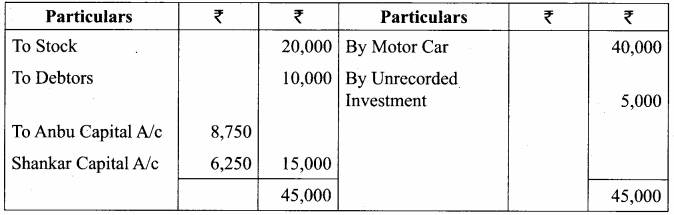Capital Account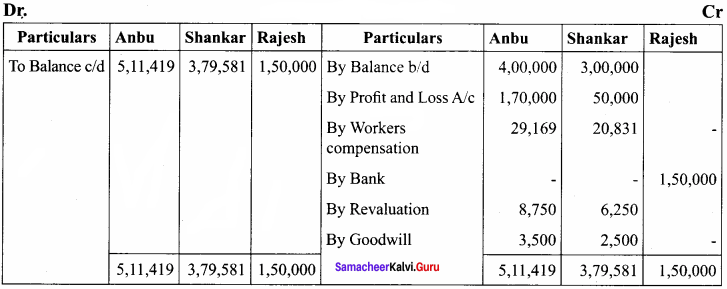Balance Sheet as on 31.03.2018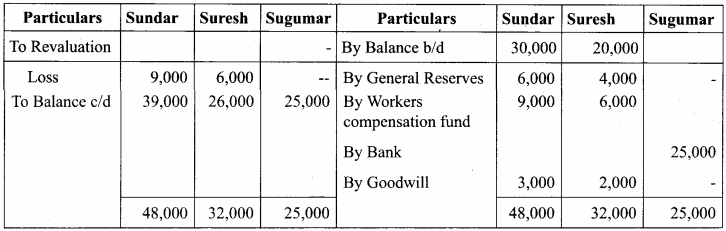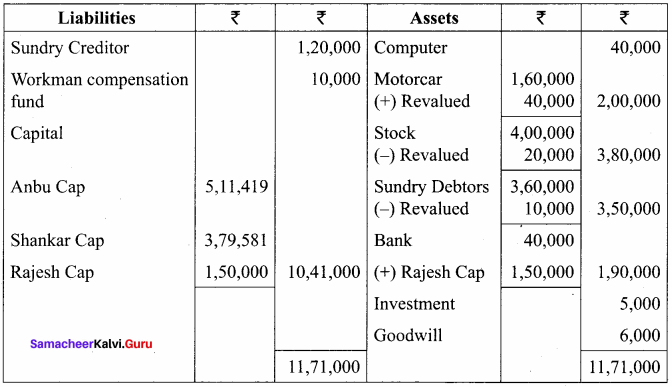Question 1.
At the time of admission of a partner calculation of new profit ratio is ………………
(a) not necessary
(b) necessary
(c) optional
(b) necessary

Question 2.
In admission, undistributed profit or loss transferred to ………………
(a) New Partners only
(b) Old Partners only
(c) All the Partners
(b) Old Partners only

Question 3.
To get sacrificing ratio should be deducted from old share ………………
(a) Gaining share
(b) New share
(c) Neither of the two
(b) new share

Question 4.
A person who is admitted to the firm is known as ………………
(a) Outgoing partners
(b) Incoming partner
(c) Both
(b) incoming partner

Question 5.
At the time of admission of a new partner the following are revalued ………………
(a) Assets
(b) Liabilities
(c) Both
(c) Both

Question 6.
New profit ratio is calculated at the time of admission, by deducting ………………
(a) Sacrifice from the old ratio
(b) Old ratio from the sacrifice
(c) Sacrifice from the new ratio
(a) Sacrifice from the old ratio

Question 7.
On the admission of a new partner ………………
(a) Old firm has to be dissolved
(b) Old partnership has to be dissolved
(c) Both old firm and partnership have to be dissolved
(d) Neither partnership nor firm has to be dissolved
(b) Old partnership has to be dissolved

Question 8.
When a new partner brings his share of goodwill in cash, the amount is debited to ………………
(b) Cash A/c
(c) Capital A/c of old partner
(d) Capital A/c of new partner